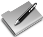• # Angular JS Expression

Posted on December 19, 2015 by Hamidul Islam in Angular JS.In angular JS, expression starts with {{ and ends with }}. So an angular JS expression looks like {{expression}}. In this tutorial we will look into various examples of Angular JS Expressions.

## Example1:Adding two numbers. For below example the output is 7

<!DOCTYPE html>
<html lang="en-US">
<body>
<div ng-app="">
{{5+2}}
</div>
</body>
</html>

## Example2:Initialize variables before using in the expression

<!DOCTYPE html>
<html lang="en-US">
<body>
<div ng-app="" ng-init="firstName='Hamidul';lastName='Islam'">
Full Name is:{{firstName + " "+lastName}}
</div>
</body>
</html>

Explanation: In the above example the firstName and lastName are initialized.  The output of the above example is

Full Name is:Hamidul Islam

## Example3:Addition and Multiplication of two numbers

<!DOCTYPE html>
<html lang="en-US">
<body>
<div ng-app="" ng-init="number1=3;number2=5">
Addition of the two numbers:{{number1+number2}}
<br/>
Multiplication of the two numbers:{{number1*number2}}
</div>
</body>
</html>

The output of the above example is

Addition of the two numbers:8
Multiplication of the two numbers:15

## Example4:Using object in expression

<!DOCTYPE html>
<html lang="en-US">
<body>
<div ng-app="" ng-init="customer={firstName:'Jan',lastName:'Marc'}">

<p>Customer last name is {{ customer.lastName }}</p>

</div>
</body>
</html>

In the above example the customer is JSON object which is initialized using ng-init.The output is

Customer last name is Marc

## Example5:Using array in expression

<!DOCTYPE html>
<html lang="en-US">
<body>
<div ng-app="" ng-init="numbers=[10,11,190,20,44]">

<p>The third number is {{ numbers }}</p>

</div>
</body>
</html>

In the above example numbers is an array which contains some numbers. The output is

The third number is 190

Post Tagged with ,

This site uses Akismet to reduce spam. Learn how your comment data is processed.

Top

By continuing to use the site, you agree to the use of cookies. more information

The cookie settings on this website are set to "allow cookies" to give you the best browsing experience possible. If you continue to use this website without changing your cookie settings or you click "Accept" below then you are consenting to this.

Close